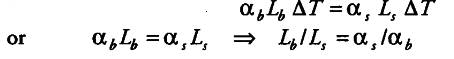# The difference between length of a certain brass rod and that of a steel rod is claimed to be constant at all temperatures. Is this possible?

Yes, it is possible to describe the difference of length to remain constant. So, the change in length of each rod must be equal at all temperature. Let L_{ b } and L_{ s } be the length of the brass and the steel rod. Let there is change in temperature \$\Delta\$T. Then,Hence, the lengths of the rods must be in the inverse ratio of the coefficient of linear expansion of their materials.